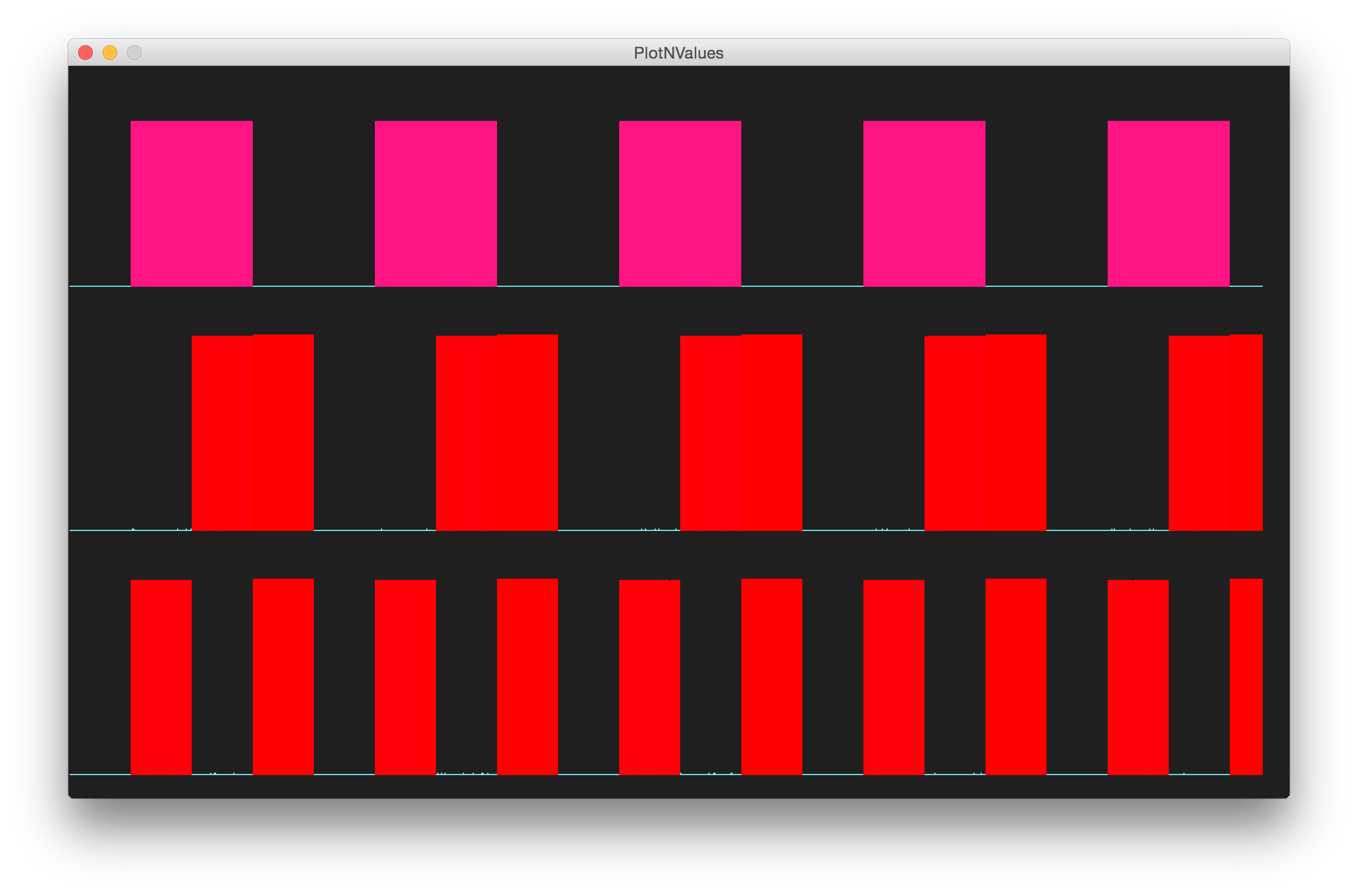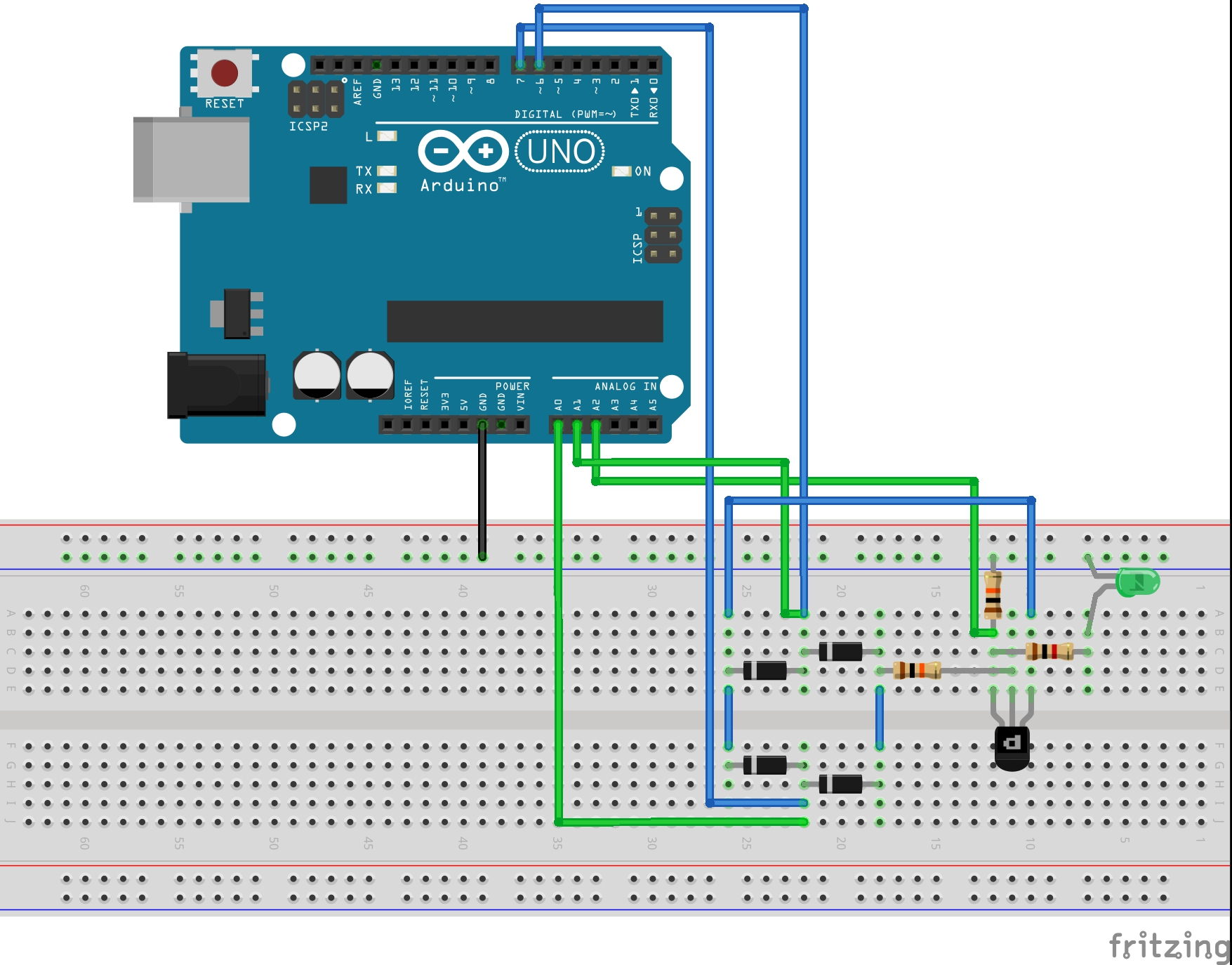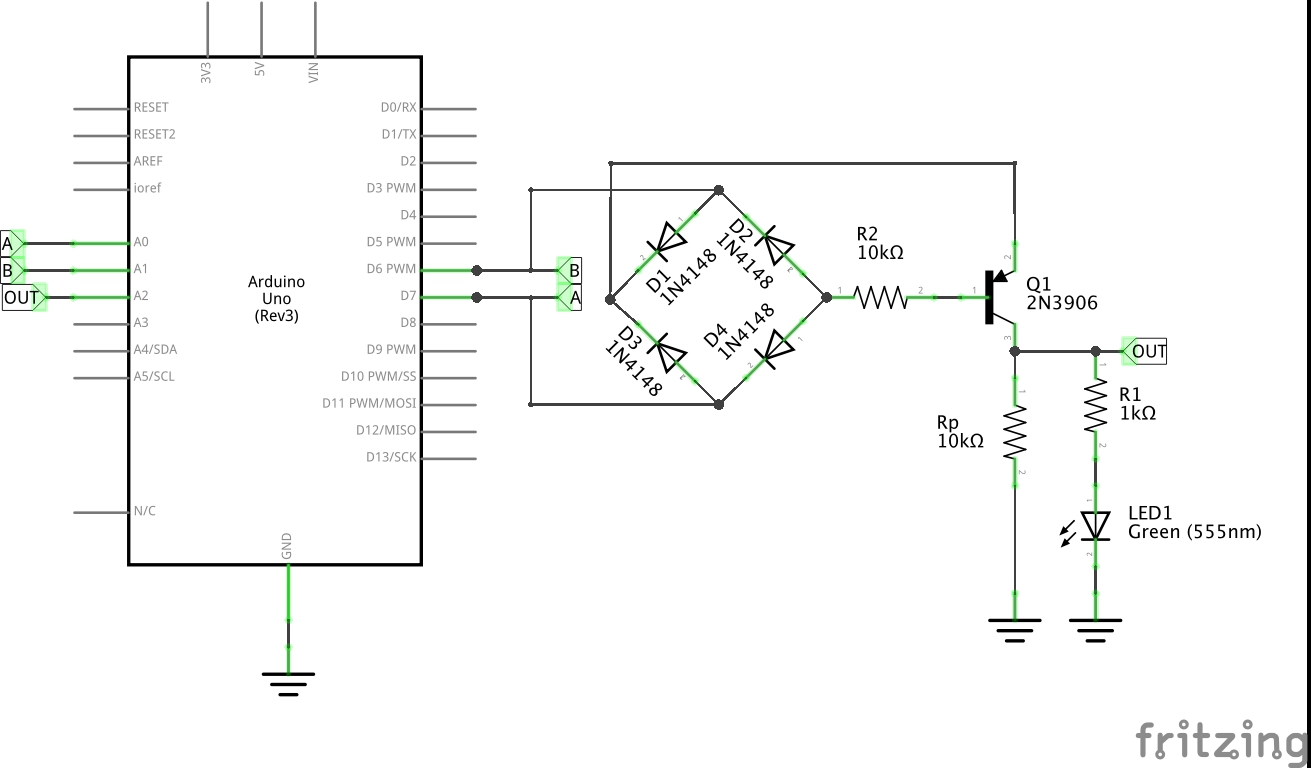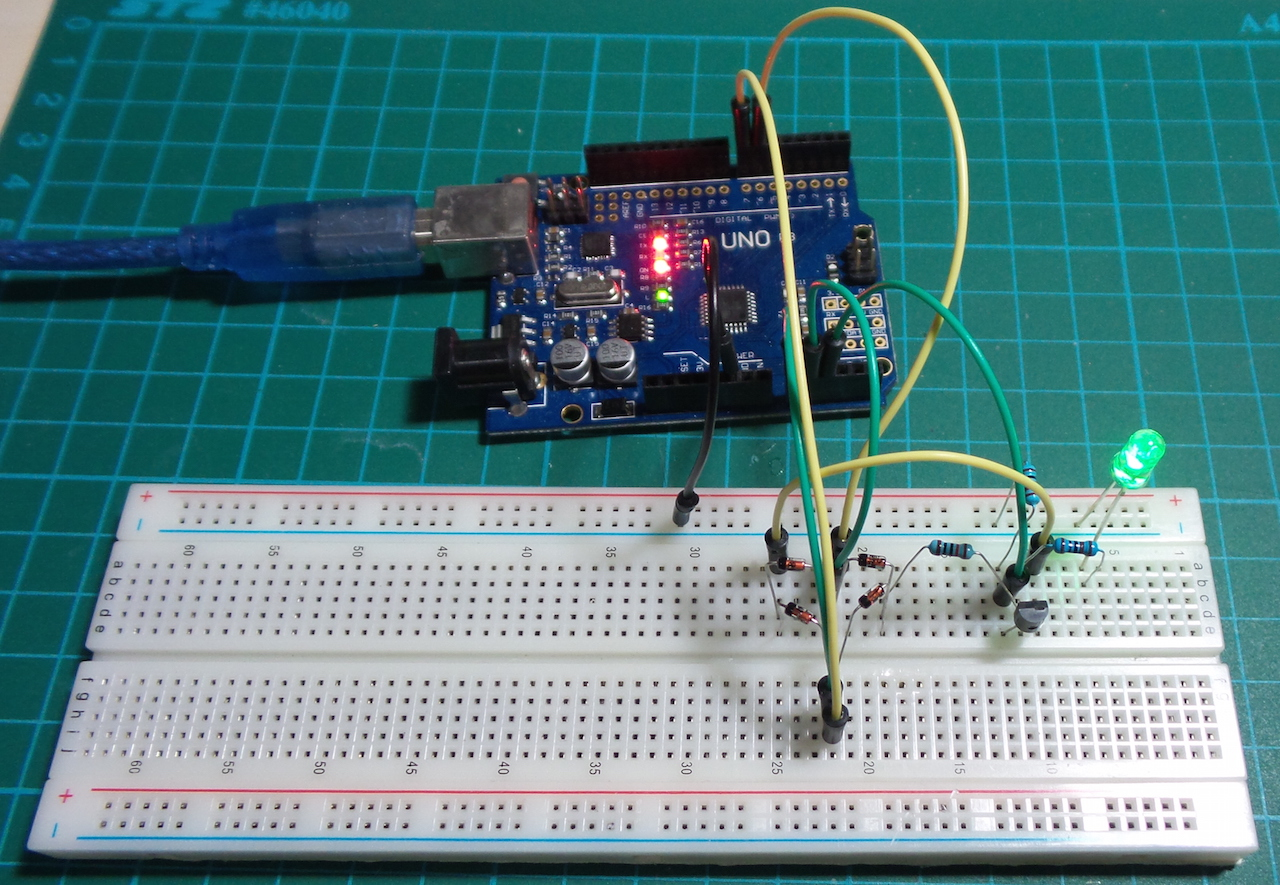# #221 ResistorTransistorLogic/XOR

Test the basic resistor-transistor logic XOR gate.Exclusive OR (XOR) operation is true only when inputs are different. Truth table:

A B OUT
0 0 0
0 1 1
1 0 1
1 1 0

An XOR may be constructed using combinatorial logic: an OR ANDed with a NAND.

This circuit uses a diode-bridge and a single transistor - quite neat. It does mean however that there is no output buffer, in fact the output is powered from the inputs.

Here’s a simulation of the circuit using Circuit Simulator by Paul Falstad.

Here’s a sample trace. The lower two traces are the signal inputs, and the upper trace is the output of the gate.The Arduino is not really a core part of the circuit. It only does the following:

• provides +5V power supply (for convenience)
• automates the toggling of the driving inputs
• measures the input and output voltages for plotting

## Construction

The circuit uses a digital output pins to sequence inputs to the gate. Three analog input pins are used to read the two input signals and the resuting output, with the values is echoed to the Arduino serial port for plotting with PlotNValues (a simple Processing sketch).